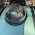## Sunday, September 21, 2014

### HMM example with depmixS4

On a scale of one to straight up voodoo, Hidden Markov Models (HMMs) are definitely up there for me.

They have all sorts of applications, and as the name suggests, they can be very useful when you wish to use a Markovian approach to represent some stochastic process.

In loose terms this just means we wish to represent our process as some set of states and probabilistic transitions between them.

For example if I am in state 1, there may be a 85% chance of staying in state 1, and a 15% chance of moving to state 2.

To complete this simple two state model, we would also have to define the transitions for state 2, namely what is the probability we will stay in state 2 if we are already in state 2, and what is the probability we will transition from state 2 to state 1.

But what if we don’t know this information? What if we just have a set of realizations from some process and a vague idea of the number of states that might be behind it?

Enter HMMs. Among other things, they can be used to determine the state transition probabilities that underlie some process, as well as the distributional parameters for each state.

That is, it can work out all the details that are "hidden" from an observer of a process. All we really need to do is specify how many states we think there are.

### Example

To test this out, and to get more familiar with the depmixS4 package, I made a small test program. It creates an observation series with:

a) a known number of states
b) known state transition matrices
c) known underlying distribution parameters

The aim is to use depmixS4 to estimate all this information, which should help get a grip on how to use the package, and also let us see if HMMs are actually any good.

We have two states, lets call them S1 and S2. They were setup with the following properties

Both states provide realizations from the normal distribution, in this case S1 has mean -1, standard deviation 1, and S2 has mean 1, standard deviation 1. We can also see the transition matrix between S1 and S2 in the last two columns.

A trajectory was sampled, and depmixS4 used to fit a HMM. It provided the following estimates

You can see it did a pretty good job of recovering the true values.

In the following plot, you can see the sample trajectory, the estimated state from the HMM and the actual state used to generate the sample. This example worked very well, it’s not always the case that things turn out so nicely.

Voodoo I tell you!

### Outro

There is no guarantee that a fitted HMM will be of any use, and even with this simple example, the state estimates can be wildly inaccurate. You can try this for yourself by using different seed values.

This example is based on one from the book Hidden Markov Models and Dynamical Systems, which I found to be an excellent resource on the topic. It is clearly written, covers the basic theory and some actual applications, along with some very illustrative examples. Source code is provided in python.

I've read (or at least tried to read) pretty much every book on HMMs I could find, and found this one to be the most useful if you are new to HMMs and are interested in applications.

You may also find these other posts about HMMs useful as well:

Fun With R and HMMs
Getting Started with Hidden Markov Models in R
Hidden Markov Models Examples in R, part 3/4

There is also the classic paper by Rabiner.

Hopefully this has shed a bit of light on HMMs and the depmixS4 package. Code is up here.#### 6 comments:

1.Excellent post. Thanks for sharing this with the R community!

1.Thank you very much, appreciate the comment!

2.Hey,

I have done a series of blogs about using HMMs for trading in R, you can see the posts at:
http://gekkoquant.com/2014/09/07/hidden-markov-models-examples-in-r-part-3-of-4/

Hope you enjoy,

Gekko

3.Thanks Pete
for this clear and brief article !

one Q:
why are the values in the Y-axis
of the Estimated and Actual State graphs,
between 1.0 and 2.0?

Sorry, I must be missing something very basic
from the example...

1.Hi, the y axis on those plots, is the state number, either 1 or 2. I got a bit lazy with the axis labels I think, the intermediate values (1.4, 1.8 etc) shouldn't really be marked. There is a slight angle to the lines as the states are sampled at discrete intervals (along the axis).

Thanks for you comment too!

4.Thank you, Pete!
Keep the simple,"real-world"
Markov and HMM examples coming...

Note: Only a member of this blog may post a comment.